# Legacy Raw I/Q Module - 8.11 English

## CPRI LogiCORE IP Product Guide (PG056)

Document ID
PG056
Release Date
2022-11-16
Version
8.11 English

This module (contained in file raw_legacy_iq_module.vhd ) is intended for users that wish to maintain the same raw interface as was present on the v1.2 core and earlier. For new designs, the regular I/Q interface is recommended. This module does not support cores implemented with the 32-bit or 64-bit datapath.

Table 3-15: Legacy Raw I/Q Module Signals - Core Interface

Port

Direction

Clock Domain

Description

iq_tx_enable

In

System Clock

Transmit enable indicating the start of a new T c

iq_tx[15:0]

Out

System Clock

IQ Transmit Data

iq_rx[15:0]

In

System Clock

basic_frame_first_word

In

System Clock

Start of new basic frame asserted once every T c

speed_select[3:0]

In

System Clock

Current Line Rate. Connect to stat_speed output of core.

Table 3-16: Legacy Raw I/Q Module Signals - Client Interface

Port

Direction

Clock Domain

Description

rx_data_valid

Out

System Clock

Asserted at the start of the basic frame, when raw_iq_rx_count is zero.

raw_iq_tx[15:0]

In

System Clock

Raw transmit I/Q data. Synchronous to clk .

raw_iq_tx_count[5:0]

Out

System Clock

Indicates position in the frame for raw transmit data.

raw_iq_rx[15:0]

Out

System Clock

Raw receive I/Q data. Synchronous to clk .

raw_iq_rx_count[5:0]

Out

System Clock

Indicates position in the frame for raw receive data.

Operation of the Raw I/Q Module at 614.4 Mb/s

This Figure illustrates transmission using the legacy raw I/Q Module at 614.4 Mb/s.

The basic frame is 8 clk periods long.

raw_iq_tx_count goes from 0 to 7.

When raw_iq_tx_count is 0, bits [7:0] in raw_iq_tx[15:0] are reserved for the control word and are ignored.

X-Ref Target - Figure 3-29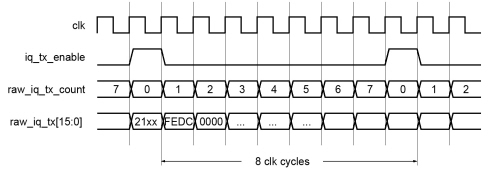When a count is presented on raw_iq_tx_count , the data is captured on the next rising clock edge. For example, in This Figure 0xFEDC is sent on count 1, 0x0000 sent on count 2, and so on. Bytes are sent out in the basic frame in the following order:

xx, 21, DC, FE, 00, 00, …

Similarly, for the receive interface at 614.4 Mb/s:

the basic frame is 8 clk periods long.

raw_iq_rx_count goes from 0 to 7.

when raw_iq_rx_count is 0, bits [7:0] in raw_iq_rx[15:0] are reserved for the basic frame control word and can be ignored.

This is illustrated in This Figure .

 X-Ref Target - Figure 3-30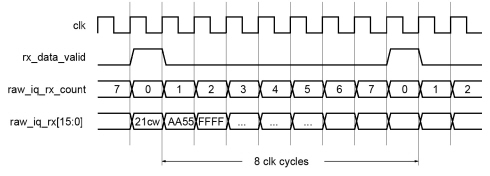When a count is presented on raw_iq_rx_count , the corresponding data is presented at the same time. For example, in This Figure 0xAA55 has been received on count 1, 0xFFFF received on count 2 and so on. Bytes are received in the basic frame in the following order:

cw, 21, 55, AA, FF, FF, …

Operation of the Legacy Raw I/Q Module at 1,228.8 Mb/s

This Figure illustrates transmission using the Legacy Raw I/Q Module at 1,228.8 Mb/s.

The basic frame is 16 clk periods long.

raw_iq_tx_count goes from 0 to 15.

When raw_iq_tx_count is 0, all bits in raw_iq_tx are reserved for the control word and are ignored.

 X-Ref Target - Figure 3-31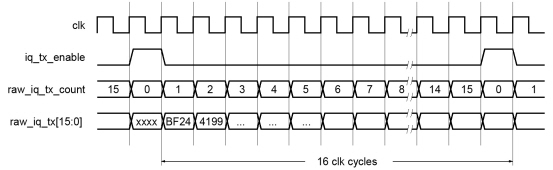When a count is presented on raw_iq_tx_count , the data is captured on the next rising clock edge. For example, in This Figure 0xBF24 is sent on count 1, 0x4199 sent on count 2, and so on. Bytes in this example are send out in the order:

xx, xx, 24, BF, 99, 41, …

Similarly for receive at 1,228.8 Mb/s:

The basic frame is 16 clk periods long.

raw_iq_rx_count goes from 0 to 15.

when raw_iq_rx_count is 0, all bits in raw_iq_rx are reserved for the basic frame control word and can be ignored.

This is illustrated in This Figure .

 X-Ref Target - Figure 3-32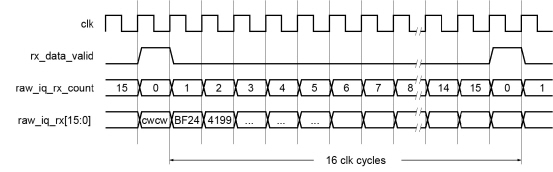When a count is presented on raw_iq_rx_count , the corresponding data is presented at the same time. For example, in This Figure 0xBF24 has been received on count 1, 0x4199 received on count 2, and so on. In this example, bytes are received in the order:

cw, cw, 24, BF, 99, 41, …

Operation of the Legacy Raw I/Q Module at 2,457.6 Mb/s

For transmitting using the Legacy Raw I/Q Module at 2,457.6 Mb/s:

the basic frame is 32 clk periods long

raw_iq_tx_count goes from 0 to 31

when raw_iq_tx_count is 0 or 1, all bits in raw_iq_tx are reserved for the control word and are ignored.

This is illustrated in This Figure .

 X-Ref Target - Figure 3-33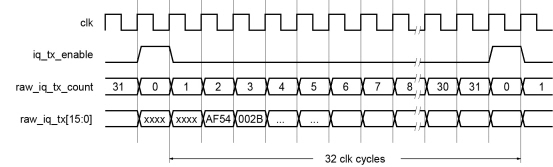When a count is presented on raw_iq_tx_count , the data is captured on the next rising clock edge. For example, in This Figure 0xAF54 is sent on count 2, 0x002B sent on count 3, and so on. In this example, bytes are sent in the order:

xx, xx, xx, xx, 54, AF, 2B, 00, …

Similarly for receive at 2,457.6 Mb/s:

the basic frame is 32 clk periods long.

raw_iq_rx_count goes from 0 to 31.

when raw_iq_rx_count is 0 or 1, all bits in raw_iq_rx are reserved for the basic frame control word and can be ignored.

This is illustrated in This Figure .

 X-Ref Target - Figure 3-34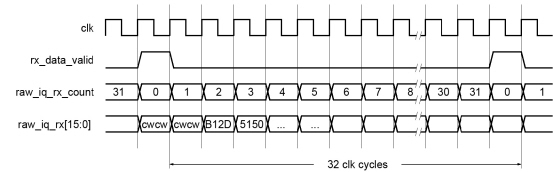When a count is presented on raw_iq_rx_count , the corresponding data is presented at the same time. For example, in This Figure 0xB12D has been received on count 1, 0x5150 received on count 2, and so on. In this example, bytes are received in the order:

cw, cw, cw, cw, 2D, B1, 50, 51, …

Operation of the Legacy Raw I/Q Module at 3,072.0 Mb/s

For transmitting using the Legacy Raw I/Q Module at 3,072.0 Mb/s:

the basic frame is 40 clk periods long.

raw_iq_tx_count goes from 0 to 39.

when raw_iq_tx_count is 0 or 1, all bits in raw_iq_tx are reserved for the control word and are ignored.

when raw_iq_tx_count is 2, bits [7:0] in raw_iq_tx are reserved for the control word and are ignored.

This is illustrated in This Figure .

 X-Ref Target - Figure 3-35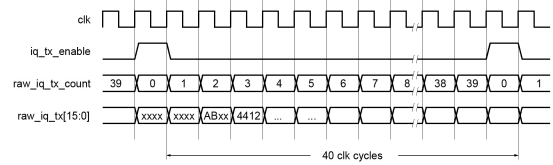When a count is presented on raw_iq_tx_count , the data is captured on the next rising clock edge. For example, in This Figure , 0xAB is sent in the second byte of count 2, 0x4412 is sent on count 3 and so on.

In this example, bytes are sent in the order:

xx, xx, xx, xx, xx, AB, 12, 44, …

Similarly for receive at 3,072.0 Mb/s:

the basic frame is 40 clk periods long.

raw_iq_rx_count goes from 0 to 39.

when raw_iq_rx_count is 0 or 1, all bits in raw_iq_rx are reserved for the basic frame control word and can be ignored.

when raw_iq_rx_count is 2, bits [7:0] in raw_iq_rx are reserved for the basic frame control word and can be ignored.

This is illustrated in This Figure .

 X-Ref Target - Figure 3-36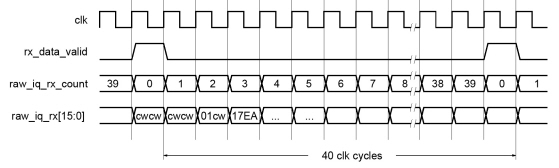When a count is presented on raw_iq_rx_count , the corresponding data is presented at the same time. For example, in This Figure , 0x01 has been received on the upper half of count 2, and 0x17EA received on count 3 and so on. In this example, bytes are received in the order:

cw, cw, cw, cw, cw, 01, EA, 17,…

Operation of the Legacy Raw I/Q Module at 4,915.2 Mb/s

For transmitting using the Legacy Raw I/Q Module at 4,915.2 Mb/s:

the basic frame is 64 clk periods long.

raw_iq_tx_count goes from 0 to 63.

when raw_iq_tx_count is 0, 1, 2 or 3, all bits in raw_iq_tx are reserved for the control word and are ignored.

This is illustrated in This Figure .

 X-Ref Target - Figure 3-37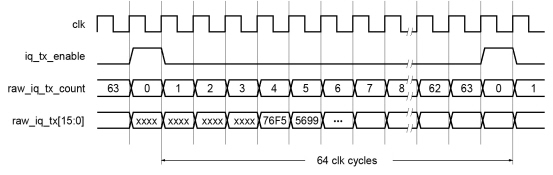When a count is presented on raw_iq_tx_count , the data is captured on the next rising clock edge. For example, in This Figure , 0x76F5 is sent on count 4, 0x5699 sent on count 5, and so on.

In this example, bytes are sent in the order:

xx, xx, xx, xx, xx, xx, xx, xx, F5, 76, 99, …

Similarly for receive at 4,915.2 Mb/s:

the basic frame is 64 clk periods long.

raw_iq_rx_count goes from 0 to 63.

when raw_iq_rx_count is 0, 1, 2 or 3 all bits in raw_iq_rx are reserved for the basic frame control word and can be ignored.

This is illustrated in This Figure .

 X-Ref Target - Figure 3-38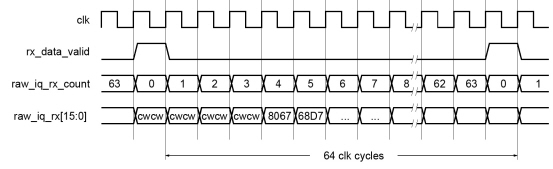When a count is presented on raw_iq_rx_count , the corresponding data is presented at the same time. For example, in This Figure , 0x8067 has been received on count 4, and 0x68D7 received on count 5 and so on. In this example, bytes are received in the order:

cw, cw, cw, cw, cw, cw, cw, cw, 67, 80, D7,…

Operation of the Legacy Raw I/Q Module at 6,144.0 Mb/s

For transmitting using the Legacy Raw I/Q Module at 6,144.0 Mb/s:

the basic frame is 80 clk periods long.

raw_iq_tx_count goes from 0 to 79.

when raw_iq_tx_count is 0, 1, 2, 3 or 4, all bits in raw_iq_tx are reserved for the control word and are ignored.

This is illustrated in This Figure .

 X-Ref Target - Figure 3-39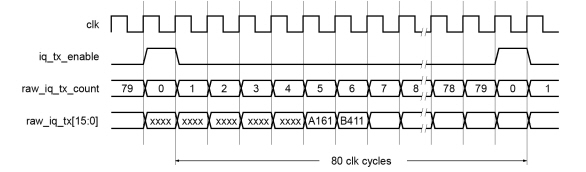When a count is presented on raw_iq_tx_count , the data is captured on the next rising clock edge. For example, in This Figure , 0xA161 is sent on count 5, 0xB411 sent on count 6, and so on. In this example, bytes are sent in the order:

xx, xx, xx, xx, xx, xx, xx, xx, xx, xx, 61, A1, 11, …

Similarly for receive at 6,144.0 Mb/s:

the basic frame is 80 clk periods long.

raw_iq_rx_count goes from 0 to 79.

when raw_iq_rx_count is 0, 1, 2, 3 or 4 all bits in raw_iq_rx are reserved for the basic frame control word and can be ignored.

This is illustrated in This Figure .

 X-Ref Target - Figure 3-40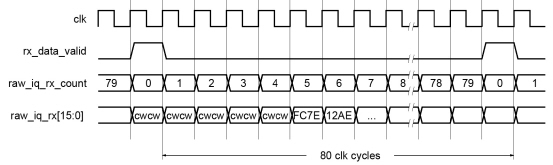When a count is presented on raw_iq_rx_count , the corresponding data is presented at the same time. For example, in This Figure , 0xFC7E has been received on count 5, and 0x12AE received on count 6 and so on. In this example, bytes are received in the order:

cw, cw, cw, cw, cw, cw, cw, cw, cw, cw, 7E, FC, AE,…